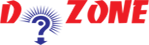﻿ Python Program to Check A Prime Number## Python Program To Check Prime Number

Here in this tutorial, we will check by using loop and if...else statement whether an integer is a prime number or not.

An integer is a prime number only if it is divisible by one or itself such as, 2, 3, 5, 7, 11, etc are prime numbers. But, 6 is not prime as it is more than 2 factors i.e. 1, 2 and 3.

``````
Python Code
# Python program to check if the input number is prime or not

num = 501

# take input from the user
# num = int(input("Enter a number: "))

# prime numbers are greater than 1
if num > 1:
# check for factors
for i in range(2,num):
if (num % i) == 0:
print(num,"is not a prime number")
print(i,"times",num//i,"is",num)
break
else:
print(num,"is a prime number")

# if input number is less than
# or equal to 1, it is not prime
else:
print(num,"is not a prime number")
Output
501 is a prime number
as it is divisible of 1 and itself
```
```

So, test the program by changing the value in the program.

## Other Python Programs

PythonTraining, 258-FF , Katewa Nagar, New Sanganer Rd, Jaipur, 302019